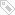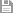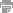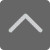# 高中数学知识点：函数

2018-11-14 07:50:34 　来源：网络整理

高中数学知识点：函数！高中数学相对于初中数学来说，难度层次更高，知识点，难点也更多，所以同学们，要想学习好高中数学，掌握好的方法是关键。下面就是小编特意为同学们整理的高中数学知识点：函数，希望对大家有所帮助。

一、高中数学函数的有关概念

1.高中数学函数函数的概念：设A、B是非空的数集，如果按照某个确定的对应关系f，使对于函数A中的任意一个数x，在函数B中都有先进确定的数f(x)和它对应，那么就称f：A→B为从函数A到函数B的一个函数.记作：y=f(x)，x∈A.其中，x叫做自变量，x的取值范围A叫做函数的定义域;与x的值相对应的y值叫做函数值，函数值的函数{f(x)|x∈A}叫做函数的值域.

注意：

函数定义域：能使函数式有意义的实数x的函数称为函数的定义域。

求函数的定义域时列不等式组的主要依据是：

(1)分式的分母不等于零;

(2)偶次方根的被开方数不小于零;

(3)对数式的真数必须大于零;

(4)指数、对数式的底必须大于零且不等于1.

(5)如果函数是由一些基本函数通过四则运算结合而成的.那么，它的定义域是使各部分都有意义的x的值组成的函数.

(6)指数为零底不可以等于零，

(7)实际问题中的函数的定义域还要保证实际问题有意义.

相同函数的判断方法：①表达式相同(与表示自变量和函数值的字母无关);②定义域一致(两点必须同时具备)

2.高中数学函数值域:先考虑其定义域

(1)观察法

(2)配方法

(3)代换法

3.函数图象知识归纳

(1)定义：在平面直角坐标系中，以函数y=f(x),(x∈A)中的x为横坐标，函数值y为纵坐标的点P(x，y)的函数C，叫做函数y=f(x),(x∈A)的图象.C上每一点的坐标(x，y)均满足函数关系y=f(x)，反过来，以满足y=f(x)的每一组有序实数对x、y为坐标的点(x，y)，均在C上.

(2)画法

A、描点法：

B、图象变换法

常用变换方法有三种

1)平移变换

2)伸缩变换

3)对称变换

4.高中数学函数区间的概念

(1)函数区间的分类：开区间、闭区间、半开半闭区间

(2)无穷区间

5.映射

一般地，设A、B是两个非空的函数，如果按某一个确定的对应法则f，使对于函数A中的任意一个元素x，在函数B中都有先进确定的元素y与之对应，那么就称对应f：AB为从函数A到函数B的一个映射。记作“f(对应关系)：A(原象)B(象)”

对于映射f：A→B来说，则应满足：

(1)函数A中的每一个元素，在函数B中都有象，并且象是先进的;

(2)函数A中不同的元素，在函数B中对应的象可以是同一个;

(3)不要求函数B中的每一个元素在函数A中都有原象。

6.高中数学函数之分段函数

(1)在定义域的不同部分上有不同的解析表达式的函数。

(2)各部分的自变量的取值情况.

(3)分段函数的定义域是各段定义域的交集，值域是各段值域的并集.

补充：复合函数

如果y=f(u)(u∈M),u=g(x)(x∈A),则y=f[g(x)]=F(x)(x∈A)称为f、g的复合函数。

二.高中数学函数的性质

1.函数的单调性(局部性质)

(1)增函数

设函数y=f(x)的定义域为I，如果对于定义域I内的某个区间D内的任意两个自变量x1，x2，当x1

如果对于区间D上的任意两个自变量的值x1，x2，当x1f(x2)，那么就说f(x)在这个区间上是减函数.区间D称为y=f(x)的单调减区间.

注意：函数的单调性是函数的局部性质;

(2)图象的特点

如果函数y=f(x)在某个区间是增函数或减函数，那么说函数y=f(x)在这一区间上具有(严格的)单调性，在单调区间上增函数的图象从左到右是上升的，减函数的图象从左到右是下降的.

(3)函数单调区间与单调性的判定方法

(A)定义法：

a.任取x1，x2∈D，且x1

b.作差f(x1)-f(x2);

c.变形(通常是因式分解和配方);

d.定号(即判断差f(x1)-f(x2)的正负);

e.下结论(指出函数f(x)在给定的区间D上的单调性).

(B)图象法(从图象上看升降)

(C)复合函数的单调性

复合函数f[g(x)]的单调性与构成它的函数u=g(x)，y=f(u)的单调性密切相关，其规律：“同增异减”

注意：函数的单调区间只能是其定义域的子区间,不能把单调性相同的区间和在一起写成其并集.

8.函数的奇偶性(整体性质)

(1)偶函数

一般地，对于函数f(x)的定义域内的任意一个x，都有f(-x)=f(x)，那么f(x)就叫做偶函数.

(2)奇函数

一般地，对于函数f(x)的定义域内的任意一个x，都有f(-x)=—f(x)，那么f(x)就叫做奇函数.

(3)具有奇偶性的函数的图象的特征

偶函数的图象关于y轴对称;奇函数的图象关于原点对称.

利用定义判断函数奇偶性的步骤：

a.首先确定函数的定义域，并判断其是否关于原点对称;

b.确定f(-x)与f(x)的关系;

c.作出相应结论：若f(-x)=f(x)或f(-x)-f(x)=0，则f(x)是偶函数;若f(-x)=-f(x)或f(-x)+f(x)=0，则f(x)是奇函数.

注意：函数定义域关于原点对称是函数具有奇偶性的必要条件.首先看函数的定义域是否关于原点对称，若不对称则函数是非奇非偶函数.若对称，(1)再根据定义判定;(2)由f(-x)±f(x)=0或f(x)/f(-x)=±1来判定;(3)利用定理，或借助函数的图象判定.

9、函数的解析表达式

(1).函数的解析式是函数的一种表示方法，要求两个变量之间的函数关系时，一是要求出它们之间的对应法则，二是要求出函数的定义域.

(2)求函数的解析式的主要方法有：

1)凑配法

2)待定系数法

3)换元法

4)消参法

10.函数较大(小)值(定义见课本p36页)

a.利用二次函数的性质(配方法)求函数的较大(小)值

b.利用图象求函数的较大(小)值

c.利用函数单调性的判断函数的较大(小)值：

如果函数y=f(x)在区间[a，b]上单调递增，在区间[b，c]上单调递减则函数y=f(x)在x=b处有较大值f(b);

如果函数y=f(x)在区间[a，b]上单调递减，在区间[b，c]上单调递增则函数y=f(x)在x=b处有较小值f(b);

以上就是小编特意为大家整理的高中数学知识点：函数，同学们学习上如果有任何疑问，可以拨打免费咨询热线：4000—121—121，那里有专业的教育规划师会为您解答。标签：保存　｜打印　｜关闭